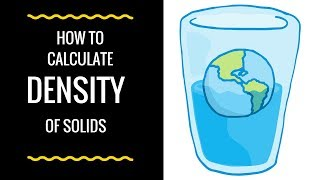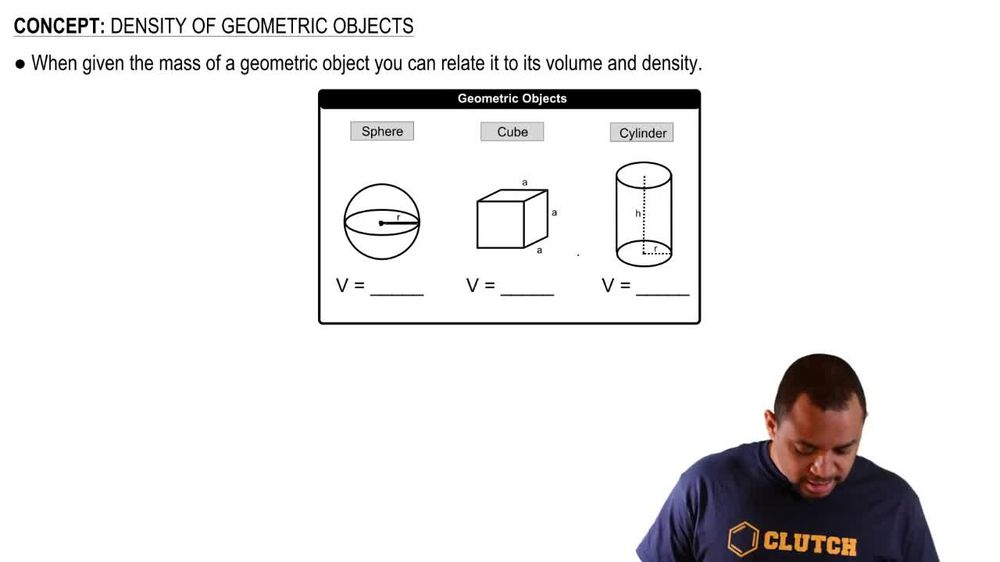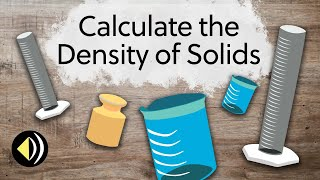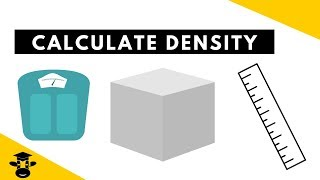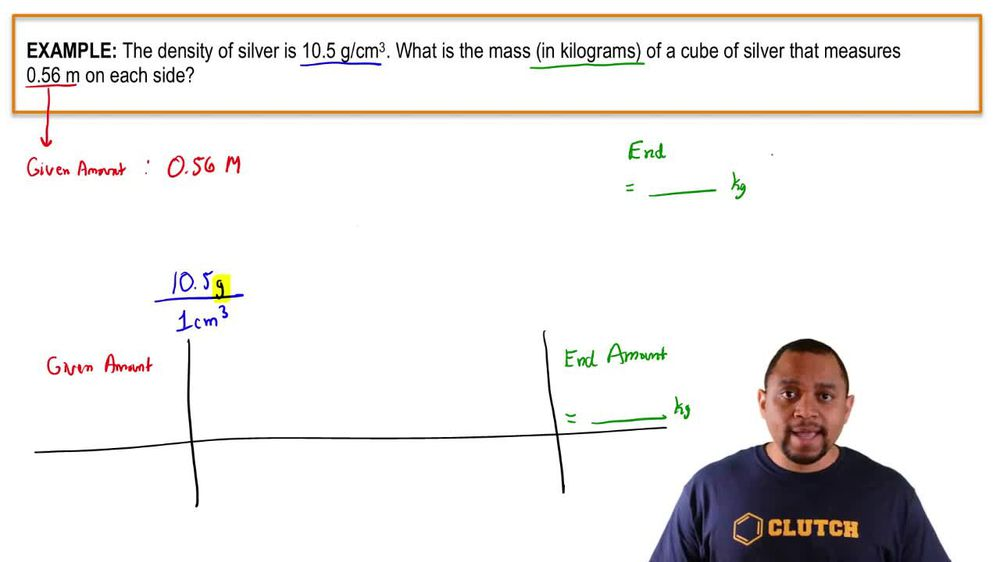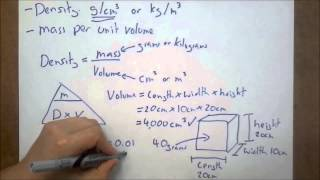Start typing, then use the up and down arrows to select an option from the list.
1. 1. Intro to General Chemistry2. Density of Geometric Objects
Problem

# Imagine that you place a cork measuring 1.30 cm * 5.50 cm * 3.00 cm in water and that on top of the cork you place a small cube of lead measuring 1.15 cm on each edge. The density of cork is 0.235 g/cm3, and the density of lead is 11.35 g/cm3. Will the combination of cork plus lead float or sink?

Relevant Solution7m
Play a video:
Hi everyone. This problem reads a sheet of styrofoam with a density of 0.12 g per cubic centimeter measuring 2.5 centimeters by six centimeters by two centimeters is placed on top of a large basin filled with water on the center of the styrofoam sheet. A cube of copper with the density of 8.96 g per cubic centimeter with an edge of one centimeter is also placed. Does the styrofoam copper combination float or sink? The density of water is one g per cubic centimeter. Okay, so what we want to determine for this problem is whether or not the styrofoam copper combination floats or sinks. Okay, so if the styrofoam copper combination ends up being the less than the density of water which were given here, the density of water is one g per cubic centimeter. If the styrofoam corporate combination is less than one g per cubic centimeter, then the combination is going to float because it's less than water. But if the density of the combination is greater than water then it's going to sink. Okay, so we need to figure out what is the average density of the styrofoam and copper together and let's recall that density is equal to mass over volume. So our goal here is to find the average density. So we're going to take the sum of the masses and the some of the volumes to figure out our average density. So based off of the problem, we have the density for both. They gave us the density for the sheet of styrofoam. And they gave us the density for the copper and were also given the volume for both. But we don't know what the mass is for both. Okay. And in order for us to figure out the average density, we need to solve for the mass. So we need to rearrange this equation so that we solve for mass. And when we rearrange it, we get mass is equal to density times volume. Alright, so let's go ahead and solve for the mass of both. We'll start off with the styrofoam. Okay, so for the styrofoam we're told the volume is 2.50 centimeters by six centimeters by two centimeters. So that gives us a volume of cubic centimeters. Okay, And we're also given the density for the styrofoam, they tell us that the density is 0.12 g per cubic centimeter. So since we know that mass is equal to density times volume, we have what we need to solve for the mass. So let's go ahead and do that. So we know the density is 0.12 g per cubic centimeter and we know the volume is 30 cubic centimeters. Alright, so looking at our units here, we see see that the cubic centimeters cancel and will be left with grams which is what we want. We want the mass. So the mass for the styrofoam is 3.60 g. Perfect. So now we know what the mass is for the styrofoam. So let's go ahead and do the same thing for the copper. Okay, so let's rewrite that. So for the copper we want to figure out its mass as well. In the problem, we're told the volume is equal to one. Well, they they tell us that the it has an edge of one centimeter. Okay, so that edge of one centimeter, we're going to cube it. So that means our volume is going to end up being one cc. Okay. And were also given the density and the problem, the density is said to be 9.698.96. Excuse me g per cubic centimeter. So now we have everything we need to solve for the mass. So we know mass is equal to density times volume. Our density is 8.96 g per cubic centimeter And the volume is one cc. Making sure our units cancel our cubic centimeters cancel. And we're left with a mass equal to 8.96 g. Okay, so now that we know what the masses for both, we want to solve for the average density. And so our average density is going to be the sum the total mass over the total volume. Because density is equal to mass over volume. So that means our total mass is equal to 3.60 g plus 8.96 g. This gives us a total mass of 12.56 g. Okay, now our total volume is equal to 30 cubic centimeters plus one cc. Which gives us a total volume of 31 cubic centimeters. So now we have what we need to solve for the average density. Okay, so the average density is going to equal the total mass over the total volume. So right that here, total mass over total volume. And we just calculated these values. So we have 12.56 g over 31 cubic centimeters. Okay, so that means our average density. So I'll just write d average is equal to 0.41 g per cubic centimeter. So we need to compare this value to our value given for the density of water. And the problem we're told the density of water is one g per cubic centimeter. So that means this average density is less than one g per cubic centimeter. Which is our density of water because it's less than one g per cubic centimeter. It's going to float because it's less dense than water. Okay, so that means out of our answer choices given it asks, does the styrofoam copper combination float or sink? Our answer is going to be answer choice a the styrofoam copper combination floats on water. Okay, so that is it for this problem? I hope this was helpful